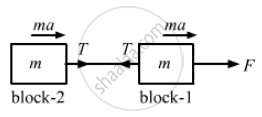# Two Blocks of Equal Mass M Are Tied to Each Other Through a Light String. One of the Blocks is Pulled Along the Line Joining Them with a Constant Force F. - Physics

Sum

Two blocks of equal mass m are tied to each other through a light string. One of the blocks is pulled along the line joining them with a constant force F. Find the tension in the string joining the blocks.

#### SolutionLet a be the common acceleration of the blocks.
For block 1,
$F - T = ma$
For block 2,
T = ma    ...(2)
Subtracting equation (2) from (1), we get:
$F - 2T = 0$
$\Rightarrow T = \frac{F}{2}$

Concept: Newton’s Second Law of Motion
Is there an error in this question or solution?

#### APPEARS IN

HC Verma Class 11, Class 12 Concepts of Physics Vol. 1
Chapter 5 Newton's Laws of Motion
Q 5 | Page 79